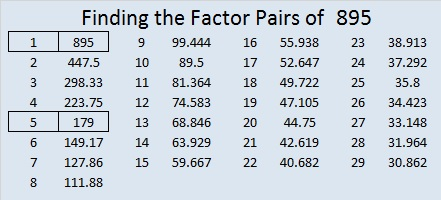# 895 and Level 6

895 is the hypotenuse of Pythagorean triple 537-716-895, which is (3-4-5) times 179.

895 is also palindrome 292 in BASE 19 because 2(19²) + 9(19) + 2(1) = 895.Print the puzzles or type the solution on this excel file: 12 factors 886-896

• 895 is a composite number.
• Prime factorization: 895 = 5 × 179
• The exponents in the prime factorization are 1 and 1. Adding one to each and multiplying we get (1 + 1)(1 + 1) = 2 × 2 = 4. Therefore 895 has exactly 4 factors.
• Factors of 895: 1, 5, 179, 895
• Factor pairs: 895 = 1 × 895 or 5 × 179
• 895 has no square factors that allow its square root to be simplified. √895 ≈ 29.91655This site uses Akismet to reduce spam. Learn how your comment data is processed.